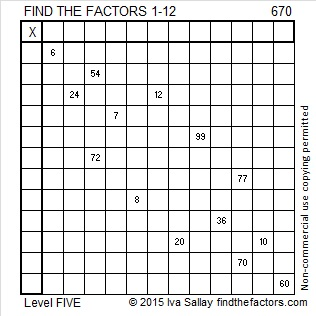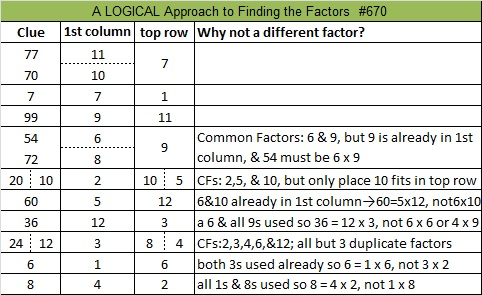# 670 and Level 5

Because 5 is one of its factors, 670 is the hypotenuse of the Pythagorean triple 402-536-670. Which factor of 670 is the greatest common factor of those three numbers?Print the puzzles or type the solution on this excel file: 12 Factors 2015-11-02

—————————————————————————————————

• 670 is a composite number.
• Prime factorization: 670 = 2 x 5 x 67
• The exponents in the prime factorization are 1, 1, and 1. Adding one to each and multiplying we get (1 + 1)(1 + 1)(1 + 1) = 2 x 2 x 2 = 8. Therefore 670 has exactly 8 factors.
• Factors of 670: 1, 2, 5, 10, 67, 134, 335, 670
• Factor pairs: 670 = 1 x 670, 2 x 335, 5 x 134, or 10 x 67
• 670 has no square factors that allow its square root to be simplified. √670 ≈ 25.884358.—————————————————————————————————Thank you, Ricardo, for tweeting the solution: